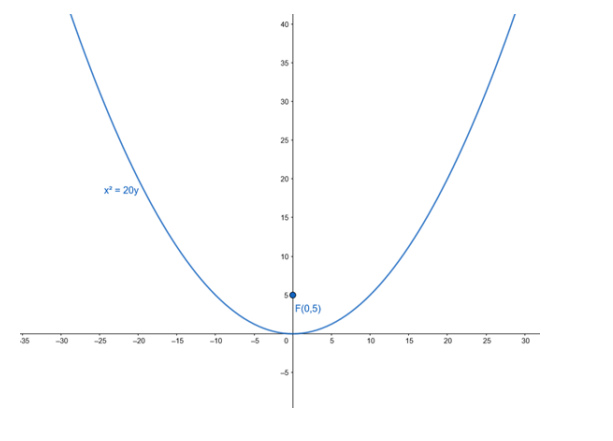# Find the equation of the parabola with vertex at the origin and focus F(0, 5)`
Question:

Find the equation of the parabola with vertex at the origin and focus F(0, 5)

Solution:

Vertex : A (0,0)

Given focus $F(0,5)$ is of the form $F(0, a)$

For Vertex $A(0,0)$ and Focus $F(0, a)$, equation of parabola is

$x^{2}=4 a y$

Here, a = 5

Therefore, equation of parabola,

$x^{2}=20 y$